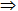# 5.4.5 - Sequence Analysis - Teradata Warehouse Miner

## Teradata Warehouse Miner User Guide - Volume 3Analytic Functions

Product
Release Number
5.4.5
Published
February 2018
Language
English (United States)
Last Update
2018-05-04
dita:mapPath
yuy1504291362546.ditamap
dita:ditavalPath
ft:empty
dita:id
tbj1499730075274

Sequence analysis is a form of association analysis where the items in an association rule are considered to have a time ordering associated with them. By default, when sequence analysis is requested, left side items are assumed to have “occurred” before right side items, and in fact the items on each side of an association rule, left or right, are also time ordered within themselves. If we use in a sequence analysis the more full notation for an association rule LR, namely {X1, X2, ...Xm}{Y1, Y2, Yn}, then we are asserting that not only do the X items precede the Y items, but X1 precedes X2, which precedes ...Xm, which precedes Y1, which precedes Y2, which precedes ...Yn.

It is important to note here that if a strict ordering of items in a sequence analysis is either not desired or not possible for some reason (such as multiple purchases on the same day), an option is provided to relax the strict ordering. With relaxed sequence analysis, all items on the left must still precede all items on the right of a sequence rule, but the items on the left and the items on the right are not time ordered amongst themselves. When the rules are presented, the items in each rule are ordered by name for convenience)

Lift and Z score are calculated differently for sequence analysis than for association analysis. Recall that the expected value of the association rule, E_LR, is given by Sup (L) * Sup (R) * N for a non-sequence association analysis. For example, if L occurs half the time and R occurs half the time, then if L and R are independent of each other it can be expected that L and R will occur together one-fourth of the time. But this does not take into account the fact that with sequence analysis, the correct ordering can only be expected to happen some percentage of the time if L and R are truly independent of each other. Interestingly, this expected percentage of independent occurrence of correct ordering is calculated the same for strictly ordered and relaxed ordered sequence analysis. With m items on the left and n on the right, the probability of correct ordering is given by “m!n!/(m + n)!”.
This is the inverse of the combinatorial analysis formula for the number of permutations of m + n objects grouped such that m are alike and n are alike.

In the case of strictly ordered sequence analysis, the applicability of the formula just given for the probability of correct ordering can be explained as follows. There are clearly m + n objects in the rule, and saying that m are alike and n are alike corresponds to restricting the permutations to those that preserve the ordering of the m items on the left side and the n items on the right side of the rule. That is, all of the orderings of the items on a side other than the correct ordering fall out as being the same permutation. The logic of the formula given for the probability of correct ordering is perhaps easier to see in the case of relaxed ordering. Since there are m + n items in the rule there are (m + n)! possible orderings of the items. Out of these, there are m! ways the left items can be ordered and n! ways the right items can be ordered while insuring that the m items on the left precede the n items on the right, so there are m!n! valid orderings out of the (m + n)! possible.

The “probability of correct ordering” factor described above has a direct effect on the calculation of lift and Z score. Lift is effectively divided by this factor, such that a factor of one half results in doubling the lift and increasing the Z score as well. The resulting lift and Z score for sequence analysis must be interpreted cautiously however since the assumptions made in calculating the independent probability of correct ordering are quite broad. For example, it is assumed that all combinations of ordering are equally likely to occur, and the amount of time between occurrences is completely ignored. To give the user more control over the calculation of lift and Z score for a sequence analysis, an option is provided to set the “probability of correct ordering” factor to a constant value if desired. Setting it to 1 for example effectively ignores this factor in the calculation of E_LR and therefore in lift and Z score.